# Fractals

To speak simple, they may be defined by one of their most important property : self similarity. In other word, each part is similar, in some sense, to the whole.
Without a serious study (introducing non integer dimensions, Hausdorff and Minkowski), we just give here a few recipes to construct,
and a little Zoo of the famous or most impressive beasts.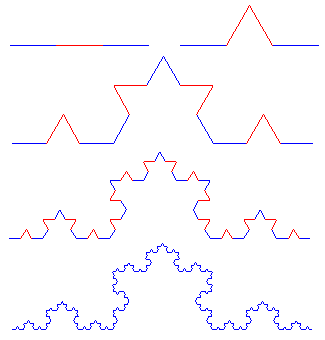#### Recursive and iteration

An intuitive way of constructing fractals is to recursively repeat on each part some transform resulting into new parts. A simple example is the Koch curve.
Starting from a segment, divide it in 3, and replace the middle segment by an equilateral "angle".
Repeat with each of the 4 new segments, and so on at infinity.
We get then a fractal "curve" (humm. getting to the limit and exact definition is let aside here...) : each part is similar to the whole curve.

The figure shows the 4 first steps. Going further, the size of bumps becomes smaller than a pixel !
This is general. Even if mathematically the recursive process must be repeated at infinity, we can just get an approximation : nobody has really seen a fractal ! Practically, after a few steps, we get a good idea of the full curve.

#### L-SystemOr "Lindenmayer system". It is the description of the rules of a "formal grammar", applied to a tortoise style drawing : Every drawn segment is defined by 'F', and a rotation by ± α as '+' or '-'

Consider the example of the Koch curve, in which α = 60°. Walking the curve, each segment 'F' has to be replaced by 'F+F--F+F'
The L-System is then defined by its initial state, named "axiom" : F and the substitution rule(s) : F → F+F--F+F

```Koch {
Angle 6  ; 1/6 turn = 60°
Axiom F
F=F+F--F+F
}
```
we get successively from the initial segment 'F'
F+F--F+F, then formally replacing each 'F' by 'F+F--F+F'
F+F--F+F + F+F--F+F -- F+F--F+F + F+F--F+F etc...

Several programs allow to draw fractals from their definition as L-system.
We get trees by using '[' and ']'.   '[' stores the current location and direction, and ']' restores that point.
Syntax details for the fractint program are given in appendix.

#### IFS

In a similar way, we can iterate a geometric transform function f of the whole plane.
The function rules will be here affine transforms, that is :;
```(x) → (a  b)(x) + (e)
(y)    (c  d)(y)   (f)
```
but it is not restricted to such functions. The condition is just that it must "reduce" the figure, that is the distance f(z)-f(z') is smaller than z-z'.

The 'S' in IFS states for "system". That is IFS = Iterated Function System We define then a set of such functions fi
The result is to find the set S of fixed points for the set of all fi. that is S = fi(S)

Practically, to draw this attractor, we start from a random point, and repeat some of the fi randomly choosen, drawing the 'final' point.
We may as well start from a fixed point, and apply a random sequence of fi up to a given depth. (each fi associated with a probability).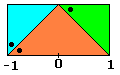Define for instance the Levy dragon.
f1 transforms the red triangle into the blue one
f2 transforms the red triangle into the green one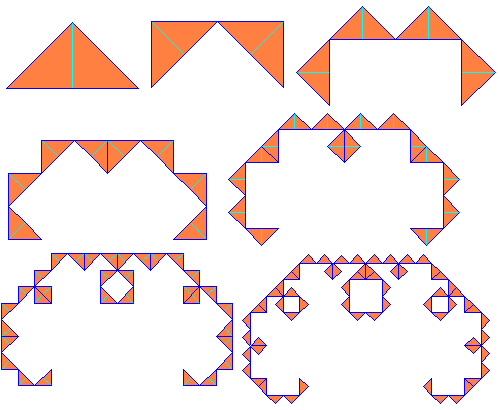```f1 : (x) = (0.5  -0.5)(x) + (-0.5)
(y)   (0.5   0.5)(y)   ( 0.5)

f2 : (x) = (0.5   0.5)(x) + (0.5)
(y)   (-0.5  0.5)(y)   (0.5)
```

Care of the orientations of the triangles ! (shown by the dot)
and finally :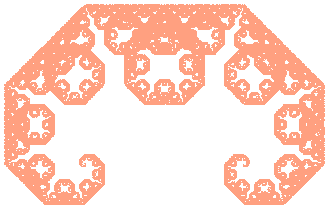This Levy dragon can even simpler be described in L-system :

```Levy {
Angle 8 ; 45°
Axiom F
F=+F--F+
}
```
Sure, we get lots of '++++++++...' in that way, that we might reduce modulo 8 (45° = 360/8), here we just spin before effectively drawing the segment ! Also '-+' = ... no rotation at all !
But the syntax doesn't allow replace rules for + and -

#### Dragons

The historical "dragon curve" has a simple L-system description
```MyDragon {
Angle 4  ; 90°
Axiom FX
X=X-YF-
Y=+FX+Y
}
```
The 'X' and 'Y' result into duplicating an half dragon by folding and rotate.
They have no direct action on the drawing operations, which just draws on 'F' and turns on the + and -

As an IFS :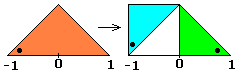```f1 : (x) = (0.5  -0.5)(x) + (-0.5)
(y)   (0.5   0.5)(y)   ( 0.5)

f2 : (x) = (-0.5  -0.5)(x) + (0.5)
(y)   (0.5   -0.5)(y)   (0.5)
```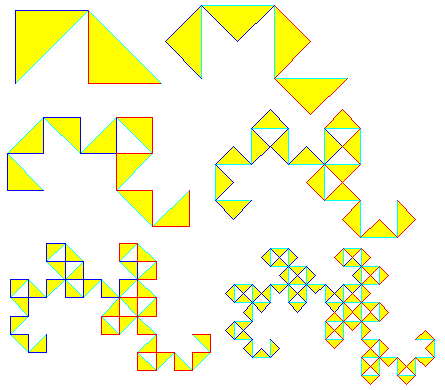We notice just a few sign changes in function f2 from the Levy dragon !
This can be seen on transforming the red triangle into the blue one (f1) and the green one (f2) Note the location of the dots !

We finally get the "Heighway dragon".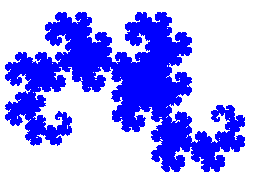But there are many other "dragons" of that kind...

#### Mandelbrot and Julia sets

One of the most powerfull way of defining fractals is through the complex plane : each point (x,y) corresponding to the complex number x + iy.
We can then do a transform z → f(z), that we can iterate. We may then consider the sequence of these iterates, depending on f and the starting point.
There may be different results :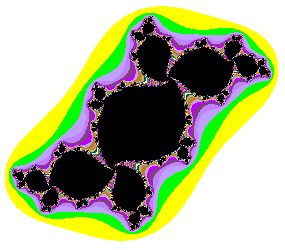• The point "goes to infinity"
• It stays "in the neighbourhood"
• It's a fixed point
We can in that way define domains in the plane.

Take as example z → z² + c, for a given c (complex)
The set of points z for which f(f(f(f(...(f(z) is limited is the Julia set for that value of c.
Really the border between the "captive" points and those who "escape".
The picture shows in black the captives (filled Julia set) for c = -0.1 + 0.7i
The colours show the points which escape to infinity, depending on the escaping speed (and of course also outside the figure).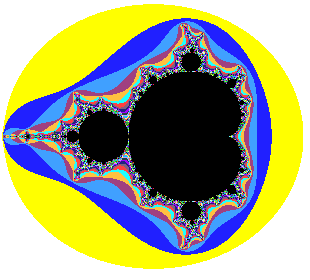The famous Mandelbrot set starts from the same iterated function z → z² + c but we consider now the values of c for which point 0 + 0i diverges to infinity, or is limited.
Here also the black points are those values of c for wich f(n)(0) is limited, and the colours are those going to infinity, depending on the escape speed.
Playing with a program which allows to zoom in selected regions of the Mandelbrot set is a cheap and exciting exploration of an always renewed universe...

#### Other fractals

There are still other fractal types, of which we say nothing more here, refer to the litterature, or explore the posssibilities of the Fractint program for instance.
Just mention without order : Henon and Lorenz attractors, the Sierpinsky gasket, chaotic branching, diffusion accretion, cellular automata etc...

#### Plants and mountains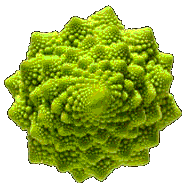Nature is full of fractal like shapes.
The coasts (of Great Britain, Norway etc. ) have been mentioned.
Of course this is only approximate, we can't go down to the atoms scale, and even further...
We must mention that the concerned "similarity" is not always that of strict geometricaly similar figures ! It can be from other parameters, as statistically distributed values.

Self similarity appears in some plants, for instance the most demonstrative "romanesco cabbage".
Some ferns also exhibit a sensible self similarity.

Hence the idea to simulate plants growth from processes constructing fractals. Of course stopping at a reasonable level.
This gives very reallistic imaginary plants.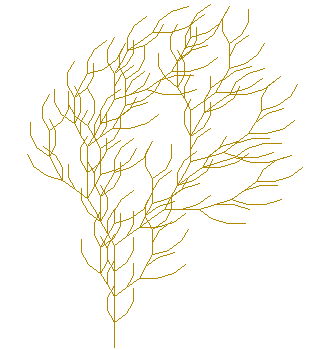This "fern" and this "tree" are drawn with just a few lines in IFS and in L-system.
In a similar way, from processes based upon self similarity, we can draw reallistic mountains, landscapes and clouds.
As natural objets are often autosimilar, some efficient image compression algorithms have been designed with that process.
We can even compose "music" (!) from autosimilarity rules.
We'll stop here.

# Appendix

A few programs to draw fractals

#### On line applets

ifs-tools Java applets to draw fractals from IFS
Ifs another
Chaos game one more time

L-system the same with L-systems
L-systems yet another
L-systems and one more

#### Others

Fractint the reference program.
detailed doc is here

IFS Construction Kit

And also others but costly, or shareware...
The only ones I have tested are those above.

A little fractal Zoo (with IFS codes)